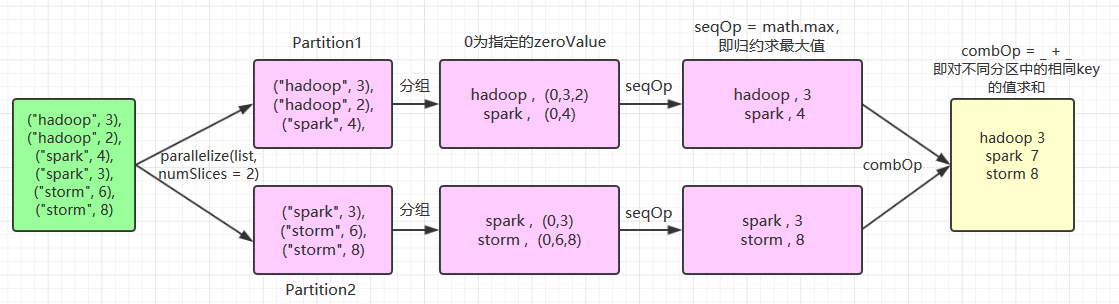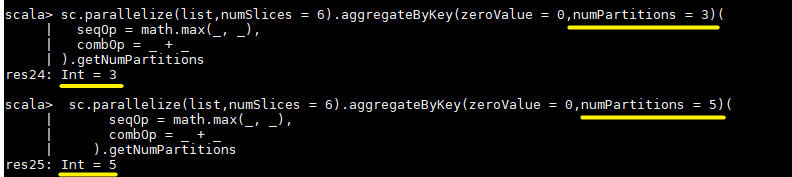# 大数据基础---Spark_Transformation和Action算子

## 一、Transformation

spark 常用的 Transformation 算子如下表：

Transformation 算子 Meaning（含义）
map(func) 对原 RDD 中每个元素运用 func 函数，并生成新的 RDD
filter(func) 对原 RDD 中每个元素使用func 函数进行过滤，并生成新的 RDD
flatMap(func) 与 map 类似，但是每一个输入的 item 被映射成 0 个或多个输出的 items（ func 返回类型需要为 Seq ）。
mapPartitions(func) 与 map 类似，但函数单独在 RDD 的每个分区上运行， func函数的类型为 Iterator<T> => Iterator<U> ，其中 T 是 RDD 的类型，即 RDD[T]
mapPartitionsWithIndex(func) 与 mapPartitions 类似，但 func 类型为 (Int, Iterator<T>) => Iterator<U> ，其中第一个参数为分区索引
sample(withReplacement, fraction, seed) 数据采样，有三个可选参数：设置是否放回（withReplacement）、采样的百分比（fraction）、随机数生成器的种子（seed）；
union(otherDataset) 合并两个 RDD
intersection(otherDataset) 求两个 RDD 的交集
distinct([numTasks])) 去重
groupByKey([numTasks]) 按照 key 值进行分区，即在一个 (K, V) 对的 dataset 上调用时，返回一个 (K, Iterable<V>)
Note: 如果分组是为了在每一个 key 上执行聚合操作（例如，sum 或 average)，此时使用 reduceByKeyaggregateByKey 性能会更好
Note: 默认情况下，并行度取决于父 RDD 的分区数。可以传入 numTasks 参数进行修改。
reduceByKey(func, [numTasks]) 按照 key 值进行分组，并对分组后的数据执行归约操作。
aggregateByKey(zeroValue,numPartitions)(seqOp, combOp, [numTasks]) 当调用（K，V）对的数据集时，返回（K，U）对的数据集，其中使用给定的组合函数和 zeroValue 聚合每个键的值。与 groupByKey 类似，reduce 任务的数量可通过第二个参数进行配置。
sortByKey([ascending], [numTasks]) 按照 key 进行排序，其中的 key 需要实现 Ordered 特质，即可比较
join(otherDataset, [numTasks]) 在一个 (K, V) 和 (K, W) 类型的 dataset 上调用时，返回一个 (K, (V, W)) pairs 的 dataset，等价于内连接操作。如果想要执行外连接，可以使用 leftOuterJoin, rightOuterJoinfullOuterJoin 等算子。
cogroup(otherDataset, [numTasks]) 在一个 (K, V) 对的 dataset 上调用时，返回一个 (K, (Iterable<V>, Iterable<W>)) tuples 的 dataset。
cartesian(otherDataset) 在一个 T 和 U 类型的 dataset 上调用时，返回一个 (T, U) 类型的 dataset（即笛卡尔积）。
coalesce(numPartitions) 将 RDD 中的分区数减少为 numPartitions。
repartition(numPartitions) 随机重新调整 RDD 中的数据以创建更多或更少的分区，并在它们之间进行平衡。
repartitionAndSortWithinPartitions(partitioner) 根据给定的 partitioner（分区器）对 RDD 进行重新分区，并对分区中的数据按照 key 值进行排序。这比调用 repartition 然后再 sorting（排序）效率更高，因为它可以将排序过程推送到 shuffle 操作所在的机器。

### 1.1 map

val list = List(1,2,3)
sc.parallelize(list).map(_ * 10).foreach(println)

// 输出结果： 10 20 30 （这里为了节省篇幅去掉了换行,后文亦同）


### 1.2 filter

val list = List(3, 6, 9, 10, 12, 21)
sc.parallelize(list).filter(_ >= 10).foreach(println)

// 输出： 10 12 21


### 1.3 flatMap

flatMap(func)map 类似，但每一个输入的 item 会被映射成 0 个或多个输出的 items（ func 返回类型需要为 Seq）。

val list = List(List(1, 2), List(3), List(), List(4, 5))
sc.parallelize(list).flatMap(_.toList).map(_ * 10).foreach(println)

// 输出结果 ： 10 20 30 40 50


flatMap 这个算子在日志分析中使用概率非常高，这里进行一下演示：拆分输入的每行数据为单个单词，并赋值为 1，代表出现一次，之后按照单词分组并统计其出现总次数，代码如下：

val lines = List("spark flume spark",
"hadoop flume hive")
sc.parallelize(lines).flatMap(line => line.split(" ")).
map(word=>(word,1)).reduceByKey(_+_).foreach(println)

// 输出：
(spark,2)
(hive,1)
(hadoop,1)
(flume,2)


### 1.4 mapPartitions

val list = List(1, 2, 3, 4, 5, 6)
sc.parallelize(list, 3).mapPartitions(iterator => {
val buffer = new ListBuffer[Int]
while (iterator.hasNext) {
buffer.append(iterator.next() * 100)
}
buffer.toIterator
}).foreach(println)
//输出结果
100 200 300 400 500 600


### 1.5 mapPartitionsWithIndex

val list = List(1, 2, 3, 4, 5, 6)
sc.parallelize(list, 3).mapPartitionsWithIndex((index, iterator) => {
val buffer = new ListBuffer[String]
while (iterator.hasNext) {
buffer.append(index + "分区:" + iterator.next() * 100)
}
buffer.toIterator
}).foreach(println)
//输出
0 分区:100
0 分区:200
1 分区:300
1 分区:400
2 分区:500
2 分区:600


### 1.6 sample

val list = List(1, 2, 3, 4, 5, 6)
sc.parallelize(list).sample(withReplacement = false, fraction = 0.5).foreach(println)


### 1.7 union

val list1 = List(1, 2, 3)
val list2 = List(4, 5, 6)
sc.parallelize(list1).union(sc.parallelize(list2)).foreach(println)
// 输出: 1 2 3 4 5 6


### 1.8 intersection

val list1 = List(1, 2, 3, 4, 5)
val list2 = List(4, 5, 6)
sc.parallelize(list1).intersection(sc.parallelize(list2)).foreach(println)
// 输出:  4 5


### 1.9 distinct

val list = List(1, 2, 2, 4, 4)
sc.parallelize(list).distinct().foreach(println)
// 输出: 4 1 2


### 1.10 groupByKey

val list = List(("hadoop", 2), ("spark", 3), ("spark", 5), ("storm", 6), ("hadoop", 2))
sc.parallelize(list).groupByKey().map(x => (x._1, x._2.toList)).foreach(println)

//输出：
(spark,List(3, 5))
(hadoop,List(2, 2))
(storm,List(6))


### 1.11 reduceByKey

val list = List(("hadoop", 2), ("spark", 3), ("spark", 5), ("storm", 6), ("hadoop", 2))
sc.parallelize(list).reduceByKey(_ + _).foreach(println)

//输出
(spark,8)
(hadoop,4)
(storm,6)


### 1.12 sortBy & sortByKey

val list01 = List((100, "hadoop"), (90, "spark"), (120, "storm"))
sc.parallelize(list01).sortByKey(ascending = false).foreach(println)
// 输出
(120,storm)
(90,spark)
(100,hadoop)


val list02 = List(("hadoop",100), ("spark",90), ("storm",120))
sc.parallelize(list02).sortBy(x=>x._2,ascending=false).foreach(println)
// 输出
(storm,120)
(hadoop,100)
(spark,90)


### 1.13 join

val list01 = List((1, "student01"), (2, "student02"), (3, "student03"))
val list02 = List((1, "teacher01"), (2, "teacher02"), (3, "teacher03"))
sc.parallelize(list01).join(sc.parallelize(list02)).foreach(println)

// 输出
(1,(student01,teacher01))
(3,(student03,teacher03))
(2,(student02,teacher02))


### 1.14 cogroup

val list01 = List((1, "a"),(1, "a"), (2, "b"), (3, "e"))
val list02 = List((1, "A"), (2, "B"), (3, "E"))
val list03 = List((1, "[ab]"), (2, "[bB]"), (3, "eE"),(3, "eE"))
sc.parallelize(list01).cogroup(sc.parallelize(list02),sc.parallelize(list03)).foreach(println)

// 输出： 同一个 RDD 中的元素先按照 key 进行分组，然后再对不同 RDD 中的元素按照 key 进行分组
(1,(CompactBuffer(a, a),CompactBuffer(A),CompactBuffer([ab])))
(3,(CompactBuffer(e),CompactBuffer(E),CompactBuffer(eE, eE)))
(2,(CompactBuffer(b),CompactBuffer(B),CompactBuffer([bB])))



### 1.15 cartesian

val list1 = List("A", "B", "C")
val list2 = List(1, 2, 3)
sc.parallelize(list1).cartesian(sc.parallelize(list2)).foreach(println)

//输出笛卡尔积
(A,1)
(A,2)
(A,3)
(B,1)
(B,2)
(B,3)
(C,1)
(C,2)
(C,3)


### 1.16 aggregateByKey

// 为了清晰，以下所有参数均使用具名传参
val list = List(("hadoop", 3), ("hadoop", 2), ("spark", 4), ("spark", 3), ("storm", 6), ("storm", 8))
sc.parallelize(list,numSlices = 2).aggregateByKey(zeroValue = 0,numPartitions = 3)(
seqOp = math.max(_, _),
combOp = _ + _
).collect.foreach(println)
//输出结果：
(hadoop,3)
(storm,8)
(spark,7)(hadoop,3)
(storm,8)
(spark,4)


(hadoop,5)
(storm,14)
(spark,7)


aggregateByKey(zeroValue = 0,numPartitions = 3) 的第二个参数 numPartitions 决定的是输出 RDD 的分区数量，想要验证这个问题，可以对上面代码进行改写，使用 getNumPartitions 方法获取分区数量：

sc.parallelize(list,numSlices = 6).aggregateByKey(zeroValue = 0,numPartitions = 3)(
seqOp = math.max(_, _),
combOp = _ + _
).getNumPartitions## 二、Action

Spark 常用的 Action 算子如下：

Action（动作） Meaning（含义）
reduce(func) 使用函数func执行归约操作
collect() 以一个 array 数组的形式返回 dataset 的所有元素，适用于小结果集。
count() 返回 dataset 中元素的个数。
first() 返回 dataset 中的第一个元素，等价于 take(1)。
take(n) 将数据集中的前 n 个元素作为一个 array 数组返回。
takeSample(withReplacement, num, [seed]) 对一个 dataset 进行随机抽样
takeOrdered(n, [ordering]) 按自然顺序（natural order）或自定义比较器（custom comparator）排序后返回前 n 个元素。只适用于小结果集，因为所有数据都会被加载到驱动程序的内存中进行排序。
saveAsTextFile(path) 将 dataset 中的元素以文本文件的形式写入本地文件系统、HDFS 或其它 Hadoop 支持的文件系统中。Spark 将对每个元素调用 toString 方法，将元素转换为文本文件中的一行记录。
saveAsSequenceFile(path) 将 dataset 中的元素以 Hadoop SequenceFile 的形式写入到本地文件系统、HDFS 或其它 Hadoop 支持的文件系统中。该操作要求 RDD 中的元素需要实现 Hadoop 的 Writable 接口。对于 Scala 语言而言，它可以将 Spark 中的基本数据类型自动隐式转换为对应 Writable 类型。(目前仅支持 Java and Scala)
saveAsObjectFile(path) 使用 Java 序列化后存储，可以使用 SparkContext.objectFile() 进行加载。(目前仅支持 Java and Scala)
countByKey() 计算每个键出现的次数。
foreach(func) 遍历 RDD 中每个元素，并对其执行fun函数

### 2.1 reduce

 val list = List(1, 2, 3, 4, 5)
sc.parallelize(list).reduce((x, y) => x + y)
sc.parallelize(list).reduce(_ + _)

// 输出 15


### 2.2 takeOrdered

def takeOrdered(num: Int)(implicit ord: Ordering[T]): Array[T] = withScope {
.........
}


// 继承 Ordering[T],实现自定义比较器，按照 value 值的长度进行排序
class CustomOrdering extends Ordering[(Int, String)] {
override def compare(x: (Int, String), y: (Int, String)): Int
= if (x._2.length > y._2.length) 1 else -1
}

val list = List((1, "hadoop"), (1, "storm"), (1, "azkaban"), (1, "hive"))
//  引入隐式默认值
implicit val implicitOrdering = new CustomOrdering
sc.parallelize(list).takeOrdered(5)

// 输出： Array((1,hive), (1,storm), (1,hadoop), (1,azkaban)


### 2.3 countByKey

val list = List(("hadoop", 10), ("hadoop", 10), ("storm", 3), ("storm", 3), ("azkaban", 1))
sc.parallelize(list).countByKey()

// 输出： Map(hadoop -> 2, storm -> 2, azkaban -> 1)


### 2.4 saveAsTextFile

val list = List(("hadoop", 10), ("hadoop", 10), ("storm", 3), ("storm", 3), ("azkaban", 1))
sc.parallelize(list).saveAsTextFile("/usr/file/temp")


## 参考资料

RDD Programming Guide

posted @ 2020-06-26 00:00  数据驱动  阅读(140)  评论(0编辑  收藏  举报